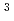The influence of the cement additives on the distribution coefficients of americium-241 to the Ca-bentonite was confirmed. The adsorption experiment of americium-241 to Ca-bentonite with cement additives was performed by the batch method, as a part of the influence evaluation of the organic in the research of TRU waste disposal. As a result, the distribution coefficient of americium-241 to the Ca-bentonite was over 1.2E+3m/kg in the condition of the absence of cement additives. In the case of low concentration (0.3g/kg) of the naphthalenesulfonic acid type cement additives, the distribution coefficient was 5.2E+2mkg. And, in the case of high concentration (30g/kg) of the same cement additives, the distribution coefficients was 2.0E-1m/kg. On the other hand in the case of flow concentration (0.5g/kg) of the polycarboxylic acid type cement additives, the distribution coefficients was over 1.3E+3m/kg. And, in the case of high concentration (50g/kg) of the same cement additives, the distribution coefficient was 1.8E-1m/kg. Here, selected cement additives concentrations were based on a standard concentration of 10g/kg when the ratio of water:cement is about one. From these results, the distribution coefficient of americium-241 to the Ca-bentonite decreases according cement additive concentration. The distribution coefficients were similar on different kinds of cement additives. The cement additives concentration influences the distribution coefficient. The distribution coefficient was small in the case of high concentration of the cement additives. That is, it is thought that the cement additives have small influences on the distribution coefficient of americium-241 to the Ca-bentonite in the case of low concentration, though the cement additives have influences on the distribution coefficient of americium-241 to the Ca-bentonite in the case of high concentration.